## Thursday, August 30, 2012

### Obtaining More Digits with the HP 50g

Intro:

I was reading the Fun With Num3ers blog by Ben Vitalis. The August 18, 2012 entry talked about certain fractions produced various patters.

Link to Ben's 10/81 post here. (8/18/12)

Examples include:

10. / 81 = 0.123456790123456790...

10 / 81 - ((3340 / 3267 * 10^-9)) = 0.12345678910111213141516... (and the number continues a counting fashion)

It was the latter fraction that inspired me to attempt to extract a larger number of decimal points. On most calculators, the most decimal points you can get is 12 in any numeric calculations. However with a calculator with CAS capabilities, we may be are able to extract more decimal places. (HP 50g, HP 49g+, TI-89, TI nSpire CAS are three models with CAS capabilities)

Steps for Fractions and Expressions - HP 50g:
1. Set the HP 50g to the Exact Mode.
2. Enter the expression. Remember, do not include a decimal point, use fractions.
4. Press EVAL.
5. Enter an integer that represents the number of decimal places. This number must be an integer!
6. Press [left shift], [EEX] (10^x) (ALOG function). Multiply.
7. Find PROPFRAC (soft key label PROPF) in the Arithmetic menu. Execute it twice. The result you want is an integer plus a fraction. The integer part represents number * 10^n. Ignore the "remainder".

Examples:

22 / 7 to 24 decimal places

n = 24

Note that 22 / 7 ≈ 3.14285714286

Keystrokes:
22 ENTER 7 / 24 10^x * PROPFRAC PROPFRAC

Press the up key and then F2 to view the answer. The result is:

3141285714285714285714285714 + 1 / 7

Hence 22 / 7 to 24 places is 3.141285714285714285714285714.

10 / 81 - ((3340 / 3267 * 10^-9)) to 24 decimal places

n = 24
10 / 81 - ((3340 / 3267 * 10^-9)) ≈ 0.12345689101

Keystrokes:
10 ENTER 81 / 3340 ENTER 3267 / 9 +/- 10^x * - 24 10^x * PROPFRAC PROPFRAC

Result:

123456789101112131415161 + (7039 / 9801)

So to 24 places, 10 / 81 - ((3340 / 3267 * 10^-9)) is 0.1234567890101112131415161.

This method can be extended to include sums and series. Finite series and sums work well. Infinite sums and series, that's another story. Sometimes a program will work better.

Steps for a program is used:
1. Set the HP 50g to the Exact Mode.
2. Enter 0. (or an appropriate starting sum)
3. Enter a program that represents a loop. The format is like this: << start end FOR var required expression + EVAL (we want exact answers) NEXT (or increment STEP >>.
4. Press EVAL twice.
5. Enter an integer that represents the number of decimal places. This number must be an integer!
6. Press [left shift], [EEX] (10^x) (ALOG function). Multiply.
7. Find PROPFRAC (soft key label PROPF) in the Arithmetic Menu. You may have to execute it twice. The result you want is an integer plus a fraction. The integer is the number * 10^n. Ignore the "remainder".

Notes:
* This method works best fast converging series. Series for π may not converge as desired (please see the example below).
* The loop can take a long time if the ending value is high.
* You have to make a mental point of where the decimal point should be. It is best to get an approximate value of the number you want to try to get more decimal points for before beginning.
* This method is not perfect! Remember, we get the result of the calculation, which may not be the desired number.

Σ (X^-1, X, 1, 20) to 24 places

Σ (X^-1, X, 1, 20) ≈ 3.5773965714

RPN syntax for Σ : variable lower_limit upper_limit expression Σ

Keystrokes, with X cleared (purged):
X ENTER 1 ENTER 20 ENTER X 1/X Σ 24 10^x * PROPFRAC PROPFRAC

Result: 10^24 * Psi(21) - 10^24 * Psi(1). Clearly, this is not what we want. Let's try a program.

1. Enter 0, then press ENTER.
2. Enter << 1 20 FOR X X INV + EVAL NEXT>>. Press ENTER, then EVAL. INV is the same as 1/X.
3. Key 24 ENTER 10^x * PROPFRAC PROPFRAC

Result:

3597739657143681911483769 + (66839 / 969969)

To 24 decimal places, Σ (X^-1, X, 1, 20) = 3.597739657143681911483769.

The difficulty for π

There are many infinite series for π. The very slow converging Gregory-Leibiniz series is the classic example:

π / 4 = 1 - 1 / 3 + 1 / 5 - 1 / 7 + 1 / 9 + ...

And the series from Nilakantha:Let's use the series from Nilakantha. Remember the integer part divided by 10^24 is our "real" answer.

Key (I combine all the steps in a single program)
<< 3
2 50 FOR K
4
K
DUP 1 +
DUP 1 +
* * INV *
-1 K 2 / 1 + ^
* + EVAL 2 STEP
24 ALOG * PROPFRAC PROPFRAC >>

Result:
3141606851347550068996630 + 1606620270334125710 / 373805630307495883

Matches π * 10^24 for the first four digits.

Changing the upper limit garners these results (ignoring the fraction):
Limit of 100: 314160685134755006899630
Limit of 250: 3141590769849795180512794
Limit of 500 (after about 20 minutes): 3141592637780581078269478

int(π *10^24) = 31415926535897932384626433

This blog entry is a technique to "trick" the calculator into giving more decimal places beyond 12

Eddie

This blog is property of Edward Shore. © 2012.

## Tuesday, August 28, 2012

### Repeated Operations - Revisited

Repeated Operations with the TI nSpire

What happens when you repeat an operation or a function on a number? I am sure we have all entered a number on a standard calculator and pressed the square root function a number of times. Eventually, each non-zero number goes towards 1.

The following graphs show are functions that are repeated. The curve in blue represents the function, the "curve" (really a Scatterplot) in red demonstrates what happens to a certain interval when I repeat a function 10 times. I used a TI nSpire CX CAS for this. That Ans function (last answer) comes in handy!

Some graphs "stabilize" after 10 iterations, and the result becomes a constant for each point in the domain. I will point that out when applicable.

Enjoy!

Eddie

This blog is property of Edward Shore. © 2012

The first set uses the domain x ∈ {0, 5}. The Scatterplot has points in this interval in increments of Δx = 0.25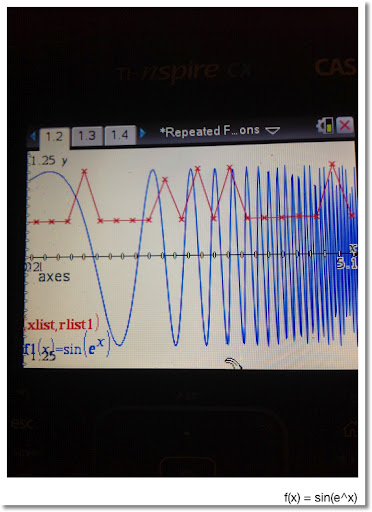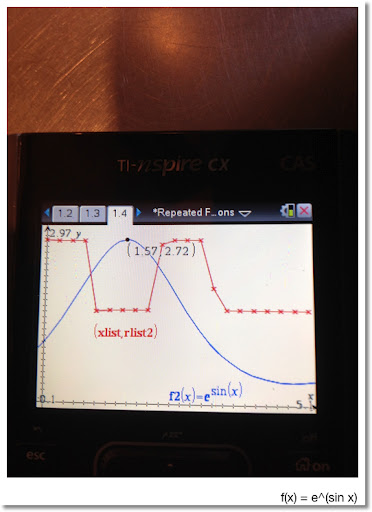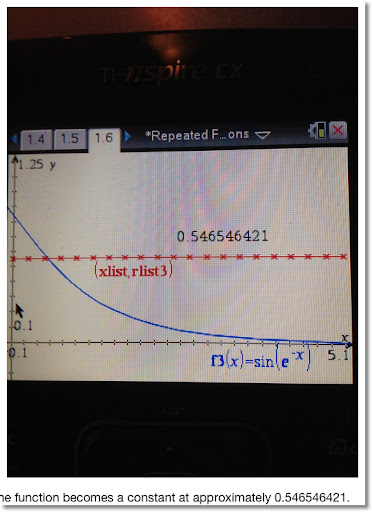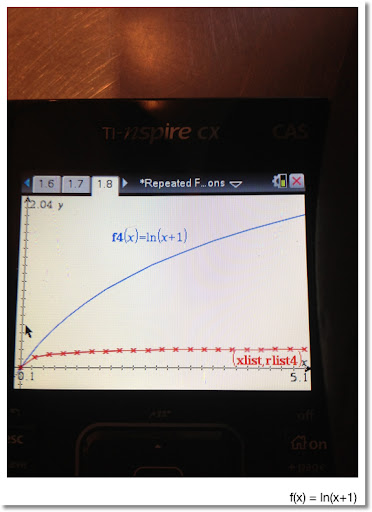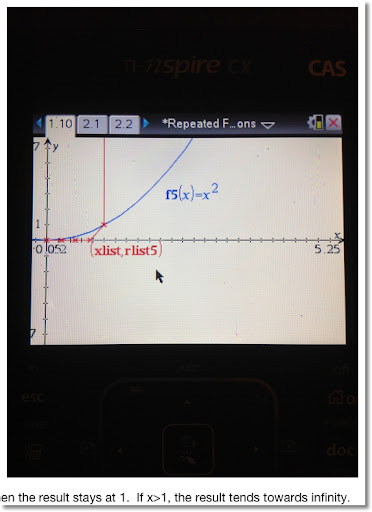The next set has the domain x ∈ [0, 10] with the Scatterplot in increments Δx = 0.1. The function is in blue, and the Scatterplot in red represents the function repeated ten times.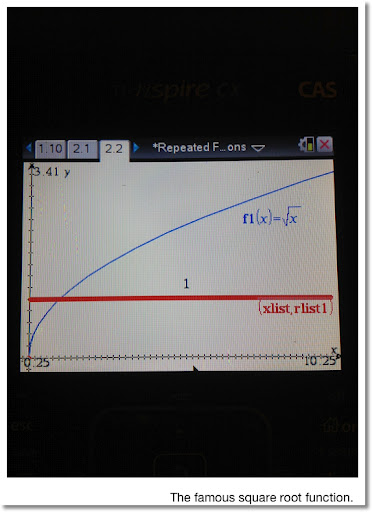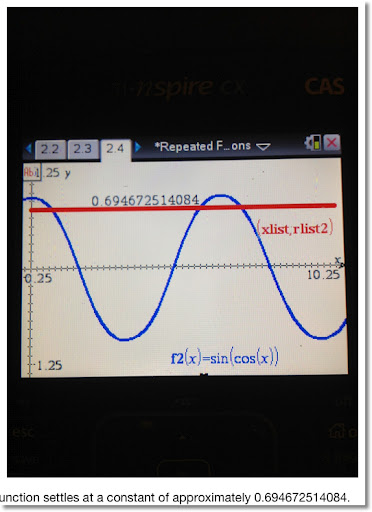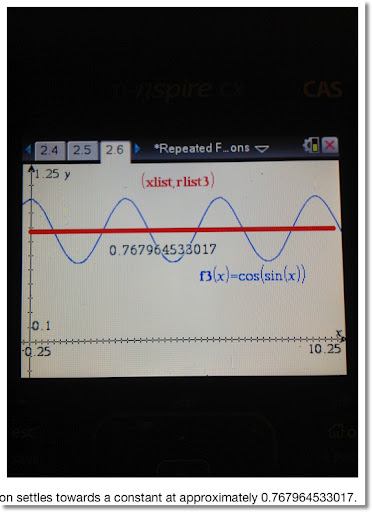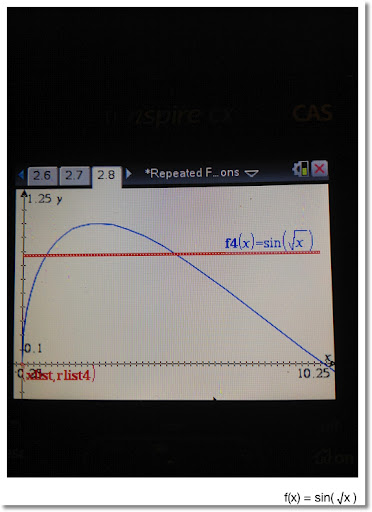### Santa Barabra

I am on vacation this week and loving it. I visited UCSB and found the math department. Below is a picture to remember my visit by.

Look forward to blogging again in September!

Eddie## Friday, August 17, 2012

### Chaos and a War Against Set Theory?

This is Eddie, at the Friends Cafe at Monrovia, CA, enjoying lunch and a great Friday.Chaos

Below are three pages from a beautiful book, "An Eye For Fractals" by Michael McGuire (1991). I got this from Book Alley in Pasadena, CA. Book Alley has every book ever written, or at least it felt like it when the aisles literally full with books.

My understanding of chaos theory and fractals is limited at this point. Making fractals involve applying repeating operations or calculations a lot (and yes this can include an infinite) of times and evaluating the results. Famous examples of fractals include the Koch Snowflake, Sierpniski Triangle (the TI-82 manual had a program to generate this), the Cantor Set, and the Barnsley Fern.

I have tried to plot the Madelbrot Set on a TI-84+, unsuccessfully.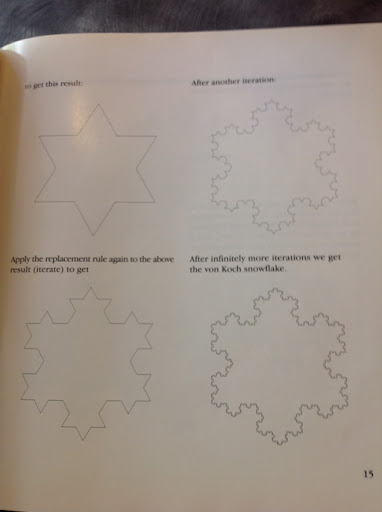Set Theory Is Evil!

Or this is what the publishers at the Christian publisher A Beka want us to think. I first heard of the publisher while I listening to episode #108 of Math/Maths Podcast. Clicking this sentence takes you to the Math/Maths podcast page. You can also download their podcast through iTunes.

They referred us to an article written by Maggie Koerth-Baker, which you can read by clicking here.

I agree with Koerth-Barker. The fundamentalist books are out of touch with reality and is a disguised as an attack on anyone and everyone who does not belong to the fundamentalist tribe. To exclude or twist facts to fit an ancient text and to teach it to children can pry the door to another Dark Age.

Mathematically, without set theory, we would be without, among other things, we would be without the ability to qualitatively compare groups of items, vector spaces, computing, and how to order objects of set. I would think that with A Beka's objection, we would not be able to tell which one of two members is greater or less - which would really screw up subtraction and the number line. However, while I was writing this blog entry, I was informed by Samuel Hansen (of the Math/Maths podcast) Twitter that their objection is to modern set - so ordering of numbers and basic math is OK. Thanks, Samuel.

Eddie

This blog is property of Edward Shore. © 2012

## Monday, August 13, 2012

### Applications and Programming: Rotation of a Graph

Rotation of a Function - TI-84+

The following program rotates the function y1(x) at an angle θ. The angle θ is measured counterclockwise, with θ = 0 indicating due east (positive x direction).

Let the coordinates (x', y') be e new coordinates after rotating the original coordiante, (x, y) at an angle θ. Then (x', y') can be calculated by the following equations:

x' = x cos θ - y sin θ
y' = x sin θ + y cos θ

The rotated coordinates are compiled into two lists: one for x', and the other for y'. A stat plot is made of the resulting lists.

Steps (TI-84+):

1. Store Y1. Two ways to do this:
* Press Y=, then edit Y1, or
* Type the function in quotes and store it in the variable Y1.

2. Run ROTATEY1 (see below). The original Y1 is graphed along its rotated sibling.

Two screen shots of examples are shown below. Have fun,

Eddie

This blog is property of Edward Shore. © 2012

Program ROTATEY1 - TI-84+

: Func
: FnOff
: PlotsOff
: FnOn 1
: PlotsOn 1
: Disp "TYPE ° FOR DEGREES"
: Input "θ:", θ
: Xmin → X
: {X cos( θ ) - Y1 sin(θ → L1
: {X sin( θ ) - Y1 cos(θ → L2
: 1→ C
: For(K,Xmin,Xmax - ΔX, ΔX)
: K + ΔX → X
: augment(L1, {X cos( θ ) - Y1 sin(θ → L1
: augment(L2, {X sin( θ ) - Y1 cos(θ → L2
: C + 1 → C
: ClrHome
: Output(1,1,"PROGRESS:
: Output(2,1,"round(100C/95,2
: End
: Plot(Scatter,L1,L2, square or marker of your choice
: DispGraph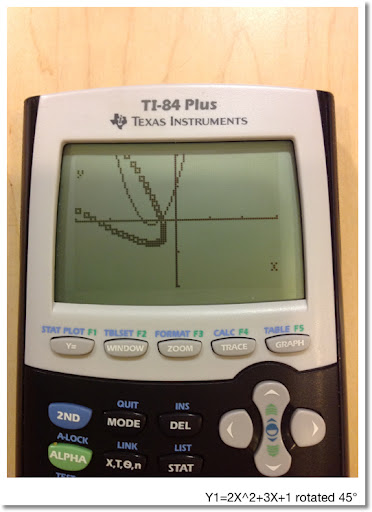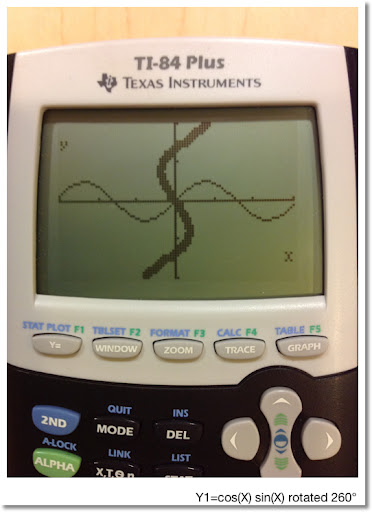## Saturday, August 4, 2012

### The Blue HP 50g Part 2 (Pictures)

First of all, this is where I bought the blue HP 50g:

The Calculator Store (Barcelona, Spain)

Now some pictures. I posted a picture of the calculator in its packaging last week. You can check that out here.

I will also be showing my black HP 50g, which I bought in 2006, and has been a companion when I was getting my Master's Degree in Mathematics.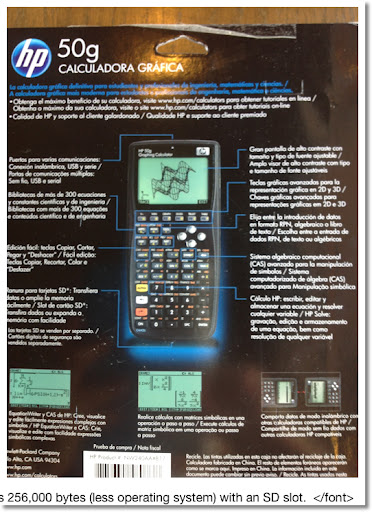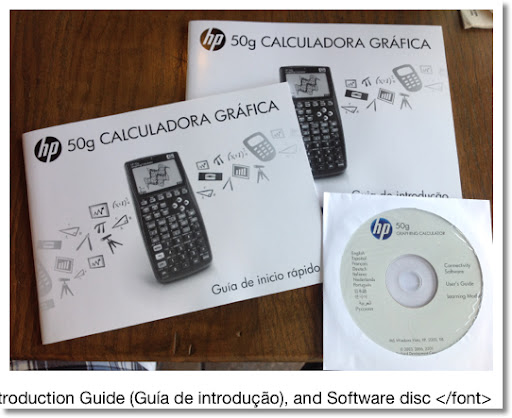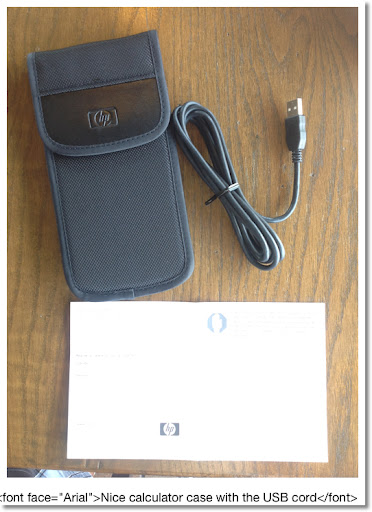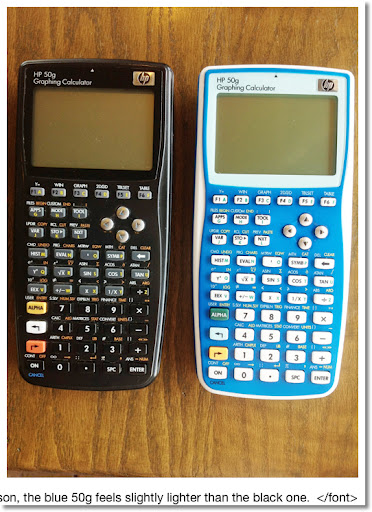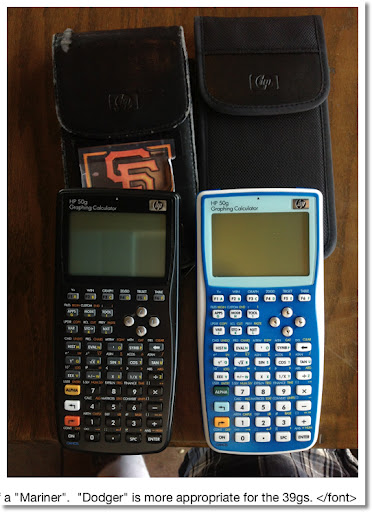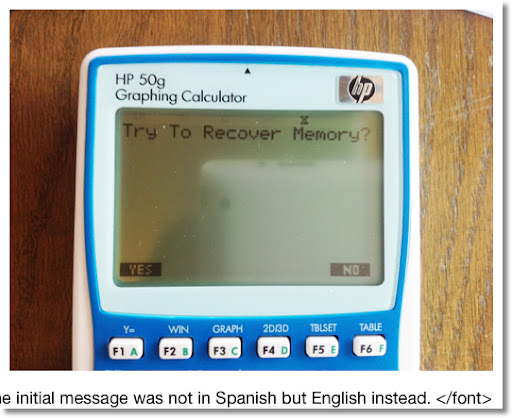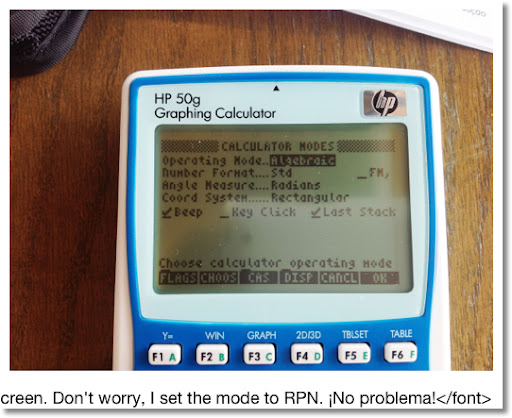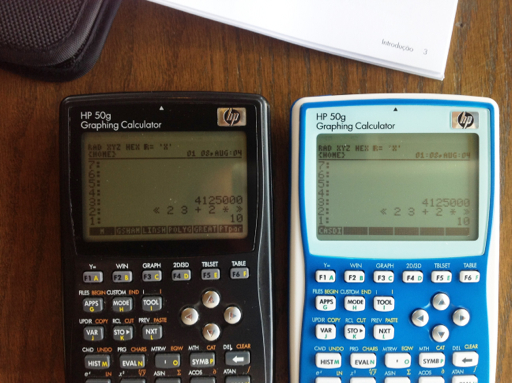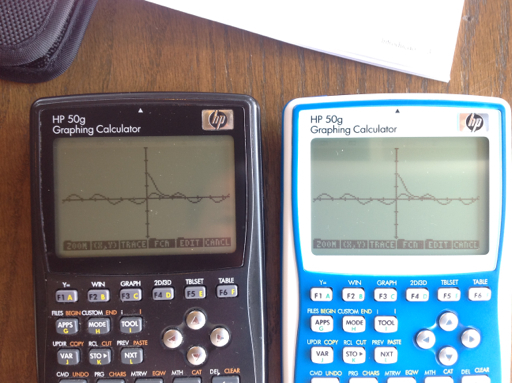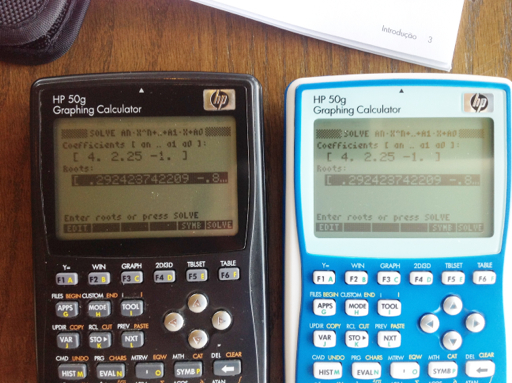Enjoy!

Eddie

This blog is property of Edward Shore. © 2012

### Applications and Programming: Ellipses

The following program works with several properties with ellipses. The general equation for an ellipse with center (x0, y0) is:

(x - x0)^2 / a^2 + (y -y0)^2 / b^2 = 1

For today's blog, I will center the ellipse at the origin (0,0).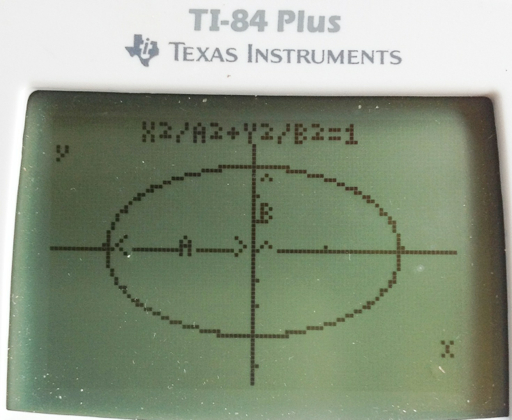With a and b as the length of the semi-axes:

* min(a,b) is the length of the semi-minor axis. min is the Minimum function, the least of a and b.
* max(a,b) is the length of the semi-major axis. max is the Maximum function, the greater of a and b.
* The eccentricity of the ellipse is e = √ (1 - [min(a,b) / max(a,b)]^2). In this sense, e is not the constant 2.7182818285... . If e = 0, then the ellipse is actually a circle. Further more, you can determine where the foci are, which is the distance e * max(a,b) along the semi-major axis.
* The area of an ellipse is easy enough: A = π a b
* The circumference is a different story. A good estimate, suggest by the NCEES, if a and b are close together is C ≈ 2 π √ ( [a^2 - b^2] / 2)

Finding the true circumference will require some calculus. Let the ellipse be defined by the parametric equations:

x(t) = a cos t
y(t) = b sin t
With 0 ≤ t ≤ 2 π

The arc length of a parametric equation is

∫ ( √ ( (dx/dt)^2 + (dy/dt)^2 ) dt, t0, t1)

Which means that the true circumference of an ellipse is

∫ ( √ ( a^2 sin(t)^2 + b^2 cos(t)^2 ) dt, 0, 2 π )

Any calculator with integration can handle this.

The ELLIPSE Program, TI-84+, 184 bytes

The following program will:
1. Prompt for a and b. (A, B)
2. Calculate eccentricity (E).
3. Calculate area (A).
4. Calculate circumference (C).
5. Draw the ellipse. The program leaves the user on the graph screen. The ellipse can be traced and used for further analysis if desired.

ELLIPSE - TI-84+

: Param
: Disp "X^2/A^2+Y^2/B^2 =1"
: Prompt A,B
: "A cos(T)"→X1T
: "B sin(T)" →Y1T
: FnOff
: PlotsOff
: FnOn 1
: 0 →Tmin
: 2 π →Tmax
: π / 128 →Tstep
: √(1-(min(A,B)/max(A,B))² → E
: π A B → R
: fnInt(√(A² sin(T)² + B² cos(T)² , T, 0, 2 π) → C
: Disp "ECC.=", E
: Pause
: Disp "AREA=", R
: Pause
: Disp "CIRC.=",C
: Pause
: ZoomFit

Here is the ELLIPSE program executed with A=2 and B=3: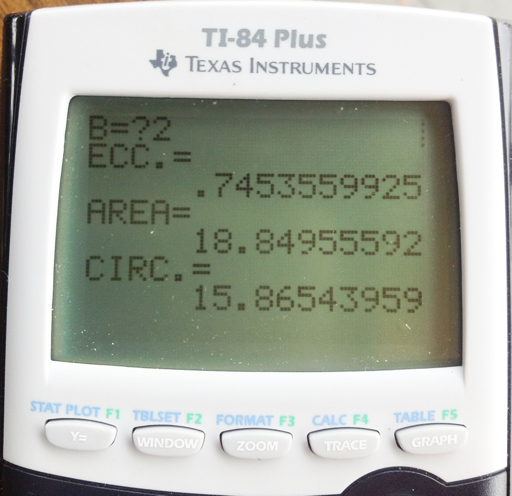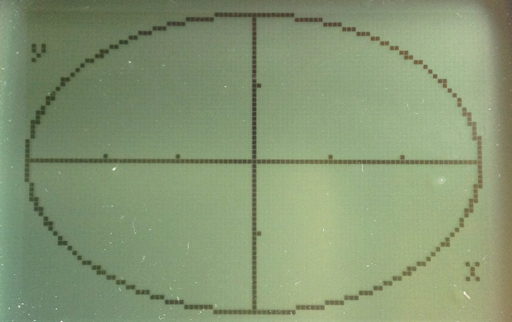I thank you once again. Thank you to the followers of my blog, love the comments, I appreciate it.

Until next time,

Eddie

This blog is property of Edward Shore. © 2012

## Thursday, August 2, 2012

### The Blue HP 50g Has Arrived

Thanks to the Calculator Store in Spain! :)

This is a HP 50g, only in a blue case.

Eddie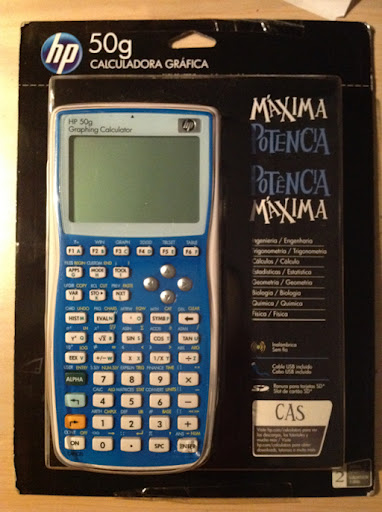### HP 15C: Error Function and Lower Tail Normal Cumulative Function

HP 15C:   Error Function and Lower Tail Normal Cumulative Function Formulas Used  Error Function  erf(x) = 2 ÷ √π * ∫( e^(-t^2) dt, t = 0 t...